# Review — AutoAugment: Learning Augmentation Strategies from Data (Image Classification)

## AutoAugment Helps to Find the Best Data Augmentation Policy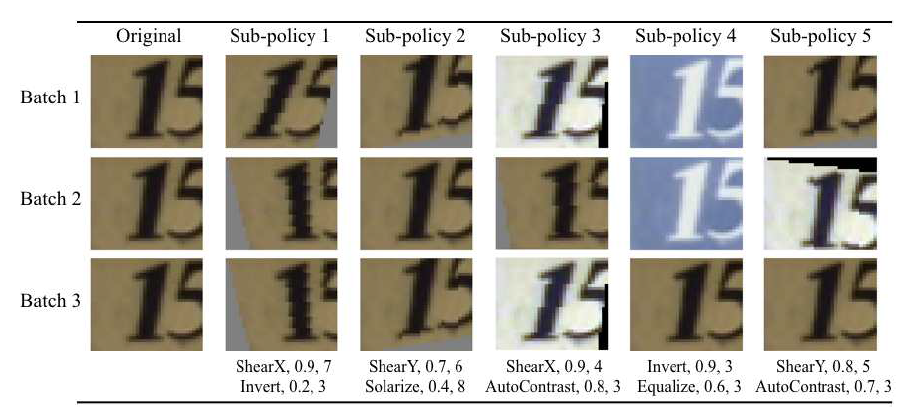One of the data augmentation policies found on SVHN

In this story, AutoAugment: Learning Augmentation Strategies from Data, (AutoAugment, AA), by Google Brain, is reviewed. In this paper:

• With AutoAugment, the augmentation policy is searched using small dataset.
• After searching the optimized augmentation policy, it is applied to train the entire dataset with higher accuracy achieved.

This is a paper in 2019 CVPR with over 600 citations. (Sik-Ho Tsang @ Medium)

# Outline

1. Search Space & Search Algorithm
2. The Controller RNN
3. Experimental Results

# 1. Search Space & Search Algorithm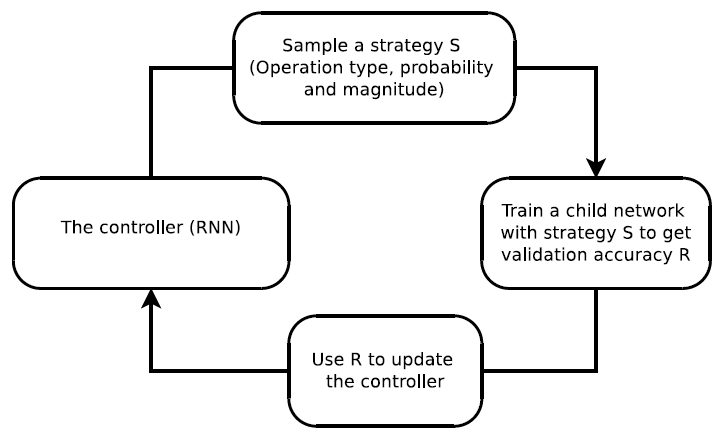Overview of the framework of using a search method (e.g., Reinforcement Learning) to search for better data augmentation policies

## 1.1. Overview

• AutoAugment consists of two components: A search algorithm and a search space.
• At a high level, the search algorithm, i.e. the controller RNN samples a data augmentation policy S, which has information about what image processing operation to use, the probability of using the operation in each batch, and the magnitude of the operation.
• The sampled policy S will be used to train a neural network with a fixed architecture, whose validation accuracy R will be sent back to update the controller.
• Since R is not differentiable, the controller will be updated by policy gradient methods.

## 1.2. Search Space Details

• In the search space, a policy consists of 5 sub-policies.
• Each sub-policy consisting of two image operations to be applied in sequence.
• Additionally, each operation is also associated with two hyperparameters: 1) the probability of applying the operation, and 2) the magnitude of the operation.
• The first figure at the top shows an example of one policy: The first sub-policy specifies a sequential application of ShearX followed by Invert. The probability of applying ShearX is 0.9, and when applied, has a magnitude of 7 out of 10.
• Then Invert is applied with probability of 0.8. The Invert operation does not use the magnitude information. These operations are applied in the specified order.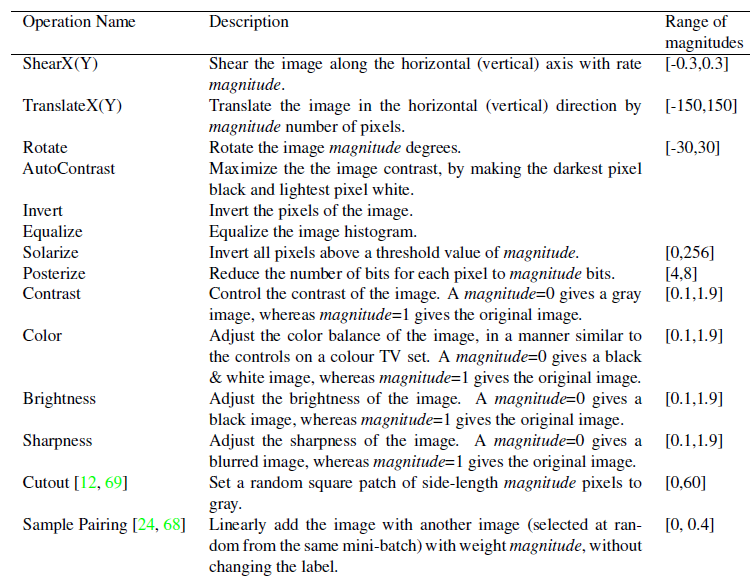Operation candidates in the search space
• Except spatial transformation operations, there are also color transformation operations, as well as some SOTA data augmentation approaches such as Cutout and mixup (Sample Pairing).
• In total, there are 16 operations in the search space, with each having its own magnitude range.
• The range of magnitudes is discretized into 10 values (uniform spacing).
• The probability of applying that operation is discretized into 11 values (uniform spacing).
• Finding each sub-policy becomes a search problem in a space of (16×10×11)² possibilities.
• The goal, however, is to find 5 such sub-policies concurrently in order to increase diversity. The search space with 5 sub-policies then has roughly (16×10×11)¹⁰≈ 2.9×1032 possibilities.

## 1.3. Search Algorithm Details

• The search algorithm that used in the experiment uses Reinforcement Learning.
• The search algorithm has two components: a controller, which is a recurrent neural network, and the training algorithm, which is the Proximal Policy Optimization algorithm .
• At each step, the controller predicts a decision produced by a softmax; the prediction is then fed into the next step as an embedding.
• In total the controller has 30 softmax predictions in order to predict 5 sub-policies, each with 2 operations, and each operation requiring an operation type, magnitude and probability.

# 2. The Controller RNN

## 2.1. The Training of the Controller RNN

• A child model is trained with augmented data generated by applying the 5 sub-policies on the training set (that does not contain the validation set).
• For each example in the mini-batch, one of the 5 sub-policies is chosen randomly to augment the image.
• The child model is then evaluated on the validation set to measure the accuracy, which is used as the reward signal to train the recurrent network controller.
• On each dataset, the controller samples about 15,000 policies.

## 2.2. Architecture of Controller RNN and Training Hyperparameters

• The controller RNN is a one-layer LSTM  with 100 hidden units at each layer and 2 × 5B softmax predictions for the two convolutional cells (where B is typically 5) associated with each architecture decision.
• Each of the 10B predictions of the controller RNN is associated with a probability.
• The joint probability of a child network is the product of all probabilities at these 10B softmaxes.
• This joint probability is used to compute the gradient for the controller RNN.
• The gradient is scaled by the validation accuracy of the child network to update the controller RNN such that the controller assigns low probabilities for bad child networks and high probabilities for good child networks.
• At the end of the search, the sub-policies are concatenated from the best 5 policies into a single policy (with 25 subpolicies).
• This final policy with 25 sub-policies is used to train the models for each dataset.

# 3. Experimental Results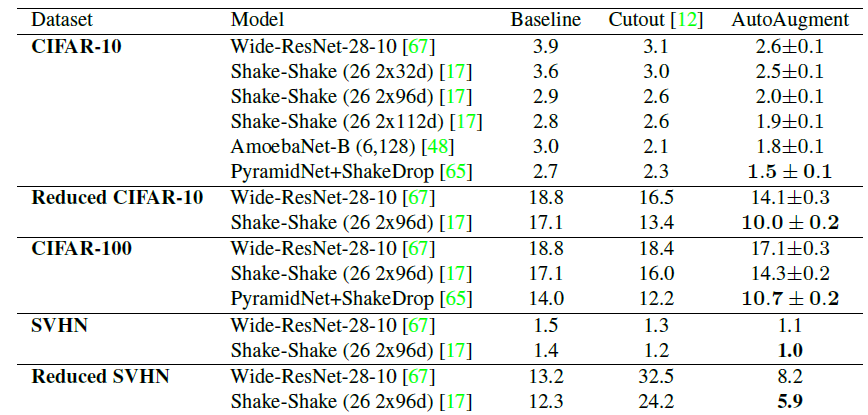Test set error rates (%) on CIFAR-10, CIFAR-100, and SVHN datasets

## 3.1. CIFAR-10

• On CIFAR-10, to search for the best policies on a smaller dataset, called “reduced CIFAR-10”, is used, which consists of 4,000 randomly chosen examples, to save time for training child network.
• As mentioned, sub-policies from the best 5 policies are concatenated to form a single policy with 25 sub-policies, which is used for all of AutoAugment experiments on the CIFAR datasets.

On CIFAR-10, AutoAugment picks mostly color-based transformations. They are are Equalize, AutoContrast, Color, and Brightness.

• Geometric transformations like ShearX and ShearY are rarely found in good policies. Furthermore, the transformation Invert is almost never applied.
• As shown above, baseline with AutoAugment, it always outperforms the baseline with AutoAugment, e.g.: WRN, Shake-Shake, AmoebaNet and PyramidNet.

## 3.2. CIFAR-100

• Similarly, AutoAugment achieve the state-of-art result on this dataset, beating the previous record of 12.19% error rate by ShakeDrop regularization.

## 3.3. SVHN

• The policies picked on SVHN are different than the transformations picked on CIFAR-10. For example, the most commonly picked transformations on SVHN are Invert, Equalize, ShearX/Y, and Rotate.
• Intuitively, this makes sense since the specific color of numbers is not as important as the relative color of the number and its background.
• Furthermore, geometric transformations ShearX/Y are two of the most popular transformations on SVHN. This also can be understood by general properties of images in SVHN: house numbers are often naturally sheared and skewed in the dataset.

## 3.4. ImageNet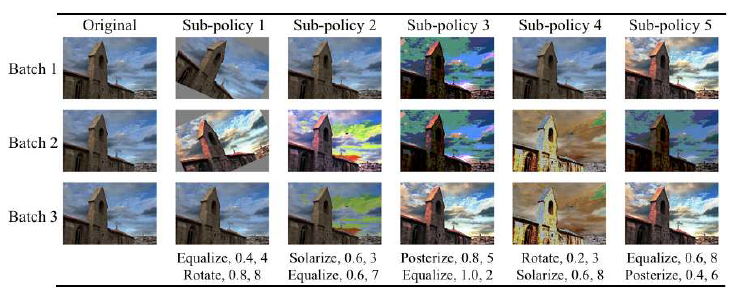One of the successful policies on ImageNet
• Most of the policies found on ImageNet used color-based transformations.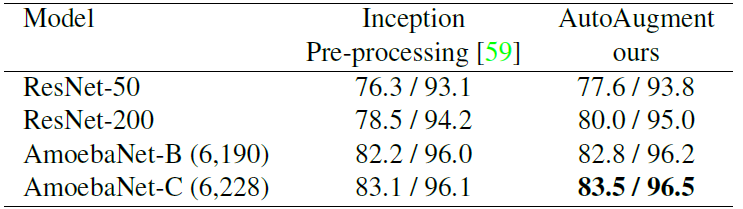Validation set Top-1 / Top-5 accuracy (%) on ImageNet
• For the baseline, the pre-processing used in GoogLeNet / Inception-v1 is used.
• As can be seen from the results, AutoAugment improves over the widely-used GoogLeNet / Inception-v1 Pre-processing.
• Secondly, applying AutoAugment to AmoebaNet-C improves its top-1 and top-5 accuracy from 83.1% / 96.1% to 83.5% / 96.5%. This improvement is remarkable given that the best augmentation policy was discovered on 5,000 images.
• The accuracy of 83.5% / 96.5% is also the new state-of-art top-1/top-5 accuracy on this dataset (without multicrop / ensembling) at that moment.

## 3.5. The Transferability of Learned Augmentation Policies to Other Datasets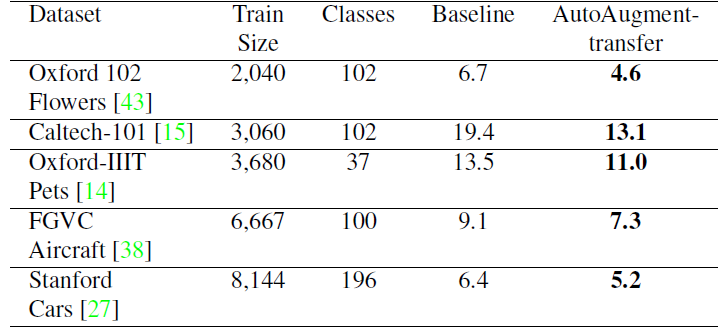Test set Top-1 error rates (%) on FGVC datasets for Inception-v4 models
• The same policy that is learned on ImageNet is used on five FGVC datasets, with image size similar to ImageNet. These datasets are challenging as they have relatively small sets of training examples while having a large number of classes.
• The above table shows that AutoAugment provides lower error rates.

There are still other experiments and ablation studies in the paper. If interested, please feel free to read the paper.

--

--